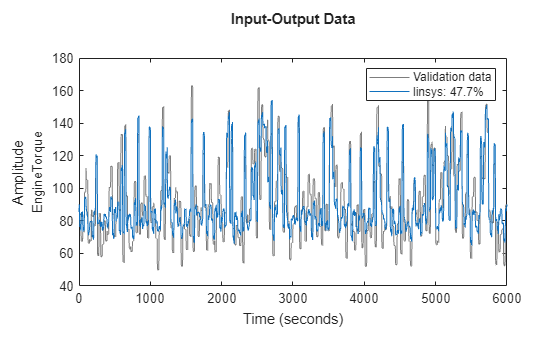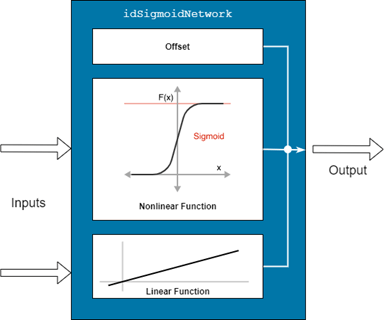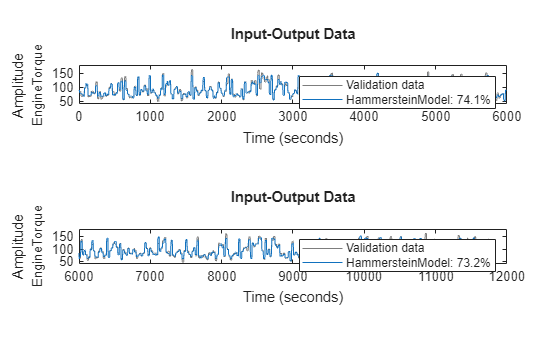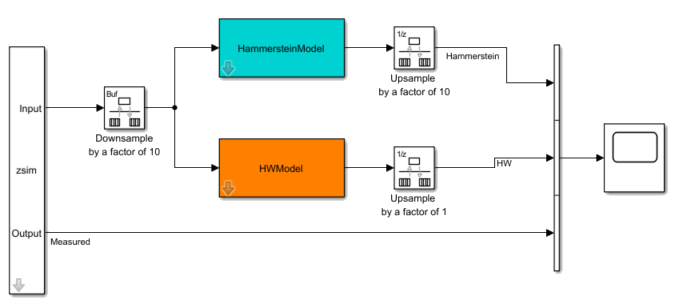# Hammerstein-Wiener Model of SI Engine Torque Dynamics

This example describes modeling the nonlinear torque dynamics of a spark-ignition (SI) engine as a Hammerstein-Wiener model. The identified model can be used for hardware-in-the-loop (HIL) testing, powertrain control, diagnostic, and training algorithm design. For example, you can use the model for aftertreatment control and diagnostics algorithm development. For more information on Hammerstein-Wiener models, see Hammerstein-Wiener Models.

You use measurements of the system inputs and outputs to identify the model. This data can come from measurements on a real engine or a high-fidelity model such as one created using the Powertrain Blockset™ SI Reference application.### Data Preparation

Load and display the engine data timetable.

```load SIEngineData IOData stackedplot(IOData) title('SI Engine Signals')```The timetable contains over one hundred thousands observations of five variables measured at 10 Hz.

• Throttle position (degrees)

• Wastegate valve area (aperture percentage)

• Engine speed (RPM)

• Spark timing (degrees)

• Engine torque (N m)

Split the data into estimation (first 60000 data points) and validation (remaining data points) portions.

```eData = IOData(1:6e4,:); % portion used for estimation vData = IOData(6e4+1:end,:); % portion used for validation```

Downsample the training data by a factor of 10. This helps speed up the model training process and also limits the focus of the fit to a lower frequency region.

```% Downsample datasets 10 times eDataD = idresamp(eData,[1 10]); vDataD = idresamp(vData,[1 10]); eDataD.Properties.TimeStep```
```ans = duration 1 sec ```

The model training objective is to generate a dynamic system that has the engine torque as output and the other four variables as inputs.

### Hammerstein-Wiener Model Identification

Hammerstein-Wiener models describe dynamic systems using one or two static nonlinear blocks in series with a linear block. The linear block is a discrete transfer function that represents the dynamic component of the model.The input and output nonlinearities can be set to flexible basis function expansions such as sigmoid or wavelet networks, or piecewise linear forms. They can also be physics-inspired forms such as saturation or deadzone. You do not have to use nonlinearities at both ends of the linear model. When the model contains only the input nonlinearity, it is called a Hammerstein model. When it contains only the output nonlinearity, it is called a Wiener model.

In this example, you identify Hammerstein-Wiener models based on different nonlinear functions. Begin by designating the input and output signals from the list of variables in the `eData` timetable.

```Inputs = ["ThrottlePosition","WastegateValve","EngineSpeed","SparkTiming"]; Output = "EngineTorque";```

The modeling approach used in this example is incremental. You first estimate a linear, four-input, one-output model for the torque dynamics. Then, you extend the linear block by adding nonlinear elements to it in a series configuration to create a Hammerstein-Wiener model.

#### Initial Linear Model

Estimate a discrete-time state-space model. The `ssest` command allows for searching for the optimal value in a given range. However, for this example, you use a fixed order of seven, which is larger than what the `ssest` order selector dialog suggests but leads to a better final nonlinear model.

Create an object to specify options for estimating state-space models, and specify the option to minimize the simulation error (instead of the prediction error) during estimation. Also specify the horizon, the mamimum number of iterations, and the order.

```ssOpt = ssestOptions(Focus="simulation"); ssOpt.N4Horizon = [15 0 26]; ssOpt.SearchOptions.MaxIterations = 200; Order = 7;```

Estimate the linear system.

```linsys = ssest(eDataD, Order, ... 'Feedthrough',true(1,4), ... 'Ts',1,... 'DisturbanceModel','none',... 'InputName',Inputs,... 'OutputName',Output,... ssOpt)```
```linsys = Discrete-time identified state-space model: x(t+Ts) = A x(t) + B u(t) + K e(t) y(t) = C x(t) + D u(t) + e(t) A = x1 x2 x3 x4 x5 x6 x7 x1 0.6307 -0.3216 0.2104 -0.0621 0.08057 0.06688 -0.4296 x2 0.2057 -0.3095 0.4587 -0.07681 0.1713 0.5015 -1.665 x3 0.02398 -0.1231 0.3411 0.7639 0.6176 0.07823 -1.12 x4 0.03332 0.04544 -0.9544 -0.2606 0.3623 -0.5218 0.1301 x5 -0.06289 -0.3276 0.2222 -0.419 0.2827 0.4606 0.1373 x6 -0.1268 0.2479 -0.1423 -0.1647 -0.169 0.2188 1.64 x7 -0.04426 -0.05109 -0.08225 0.005721 0.1281 -0.1702 1.033 B = ThrottlePosi WastegateVal EngineSpeed SparkTiming x1 -0.002453 -0.01188 0.0003596 0.003926 x2 -0.02644 -0.1191 0.003484 0.03948 x3 -0.02189 -0.1015 0.002956 0.0345 x4 0.01706 0.0783 -0.002274 -0.02633 x5 -0.0164 -0.07734 0.002247 0.02601 x6 0.01384 0.06275 -0.001798 -0.021 x7 0.001481 0.006872 -0.000192 -0.002306 C = x1 x2 x3 x4 x5 x6 x7 EngineTorque 558.3 -174.4 98.83 17.78 61.55 31.32 -375.9 D = ThrottlePosi WastegateVal EngineSpeed SparkTiming EngineTorque 0.1375 0.1187 0.0008919 0.06466 K = EngineTorque x1 0 x2 0 x3 0 x4 0 x5 0 x6 0 x7 0 Sample time: 1 seconds Parameterization: FREE form (all coefficients in A, B, C free). Feedthrough: yes Disturbance component: none Number of free coefficients: 88 Use "idssdata", "getpvec", "getcov" for parameters and their uncertainties. Status: Estimated using SSEST on time domain data "eDataD". Fit to estimation data: 46.39% FPE: 173.9, MSE: 171.7 ```

Compare the simulated response of linsys against the measured engine torque used for training.

```clf compare(eDataD, linsys)```The linear model is able to emulate the measured output to only about 50% on the Normalized Root Mean Squared Error (NRMSE) measure. However, as shown next, it provides an excellent starting point for the creation of the Hammerstein-Wiener model.

#### Hammerstein Model

Identify a model that uses a sigmoid network as input nonlinearity for each of its four inputs. A sigmoid network is a feedforward, single-hidden-layer network represented by the `idSigmoidNetwork` object.This nonlinear function is composed of parameters corresponding to the the linear term, the offset, and a nonlinear term expressed as a weighted sum of dilated and shifted sigmoid units. You have the option of disabling the linear function, the offset term, or both. You can also pick how many sigmoid units the nonlinear function employs. For this example, exclude both the linear component and the offset term, and set the number of sigmoid units to 3.

Create the sigmoid-based nonlinear function.

```NumberOfUnits = 3; % determined by trial and error NonlinearFcn = idSigmoidNetwork(NumberOfUnits,false,false)```
```NonlinearFcn = Sigmoid Network Nonlinear Function: Sigmoid network with 3 units Linear Function: not in use Output Offset: not in use Inputs: {1x0 cell} Outputs: {1x0 cell} NonlinearFcn: 'Sigmoid units and their parameters' LinearFcn: 'Linear function parameters' Offset: 'Offset parameters' ```

Create a template model (using the `idnlhw` model constructor) whose linear component is set to `linsys` and the input nonlinearity to `NonlinearFcn`.

`HammersteinModel = idnlhw(linsys, NonlinearFcn, [])`
```HammersteinModel = Hammerstein-Wiener model with 1 output and 4 inputs Linear transfer function matrix corresponding to the orders: nb = [8 8 8 8] nf = [7 7 7 7] nk = [0 0 0 0] Input nonlinearities: Input 1: Sigmoid network with 3 units Input 2: Sigmoid network with 3 units Input 3: Sigmoid network with 3 units Input 4: Sigmoid network with 3 units Output nonlinearity: None Sample time: 1 seconds Status: Created by direct construction or transformation. Not estimated. More information in model's "Report" property. ```

Inspect the linear component of the model.

`HammersteinModel.B % numerator of the linear transfer function`
```ans=1×4 cell array {[0.1375 -0.0179 -0.0559 -0.0440 -0.0480 0.0161 0.0401 -0.0222]} {[0.1187 -0.1175 0.0391 -0.1343 0.1394 -0.1045 0.1484 -0.0888]} {[8.9186e-04 -0.0025 0.0025 3.1157e-04 -0.0026 0.0042 -0.0053 0.0027]} {[0.0647 -0.0655 0.0766 -0.0850 0.0083 0.0122 -0.0368 0.0279]} ```
`HammersteinModel.F % denominator of the linear transfer function`
```ans=1×4 cell array {[1 -1.9356 1.8174 -1.7491 1.2668 -0.4497 0.0571 9.3696e-04]} {[1 -1.9356 1.8174 -1.7491 1.2668 -0.4497 0.0571 9.3696e-04]} {[1 -1.9356 1.8174 -1.7491 1.2668 -0.4497 0.0571 9.3696e-04]} {[1 -1.9356 1.8174 -1.7491 1.2668 -0.4497 0.0571 9.3696e-04]} ```

Furthermore, fix the poles of the model by freezing the coefficients of the denominator ($F\left(q\right)$) of the linear model.

`HammersteinModel.Ffree = false; % fix the denominator to its current value`

For model training, we use the `lsqnonlin` search method and do not normalize the I/O signals.

```% Configure training options nlhwopt = nlhwOptions('Display','on'); nlhwopt.SearchMethod = 'lsqnonlin'; nlhwopt.SearchOptions.MaxIterations = 300; % choose to NOT normalize the I/O signals nlhwopt.Normalize = false;```

Estimate the parameters of the template model (`HammersteinModel`) to fit the data (`eDataD`) using the `nlhw` command.

```tic HammersteinModel = nlhw(eDataD,HammersteinModel,nlhwopt)```
```HammersteinModel = Hammerstein-Wiener model with 1 output and 4 inputs Linear transfer function matrix corresponding to the orders: nb = [8 8 8 8] nf = [7 7 7 7] nk = [0 0 0 0] Input nonlinearities: Input 1: Sigmoid network with 3 units Input 2: Sigmoid network with 3 units Input 3: Sigmoid network with 3 units Input 4: Sigmoid network with 3 units Output nonlinearity: None Sample time: 1 seconds Status: Termination condition: Maximum number of iterations or number of function evaluations reached.. Number of iterations: 301, Number of function evaluations: 302 Estimated using NLHW on time domain data "eDataD". Fit to estimation data: 72.98% FPE: 44.55, MSE: 43.61 More information in model's "Report" property. ```
`toc`
```Elapsed time is 108.408758 seconds. ```

Perform a validation of the model quality by comparing its simulated response against the measured responses in the estimation and the validation datasets. Note that you check only against the decimated data here.

```subplot(211) compare(eDataD,HammersteinModel) % compare against estimation data title('Hammerstein Model: Comparison to Estimation Data') subplot(212) compare(vDataD,HammersteinModel) % compare against validation data title('Comparison to Validation Data (Downsampled)')```#### Hammerstein-Wiener Model

Identify a model that uses piecewise linear functions for both input and output nonlinearities. You have a linear model that you want to extend by adding nonlinear elements. To ensure that the identified model is no worse than the linear one, you freeze the linear block parameters (the $B\left(q\right)$ and $F\left(q\right)$ polynomials) completely.

```PWLin = idPiecewiseLinear(12); % input nonlinearities PWLout = idPiecewiseLinear(3); % output nonlinearity HWModel = idnlhw(linsys, PWLin, PWLout); % Fix the linear component completely HWModel.Bfree = false; HWModel.Ffree = false; % Configure estimation options nlhwopt = nlhwOptions('Display','on'); nlhwopt.SearchMethod = 'lm'; nlhwopt.SearchOptions.MaxIterations = 100; % Estimate the breakpoint locations of PWLin, PWLout HWModel = nlhw(eDataD,HWModel,nlhwopt)```
```HWModel = Hammerstein-Wiener model with 1 output and 4 inputs Linear transfer function matrix corresponding to the orders: nb = [8 8 8 8] nf = [7 7 7 7] nk = [0 0 0 0] Input nonlinearities: Input 1: Piecewise linear with 12 break-points Input 2: Piecewise linear with 12 break-points Input 3: Piecewise linear with 12 break-points Input 4: Piecewise linear with 12 break-points Output nonlinearity: Piecewise linear with 3 break-points Sample time: 1 seconds Status: Termination condition: Maximum number of iterations reached.. Number of iterations: 100, Number of function evaluations: 527 Estimated using NLHW on time domain data "eDataD". Fit to estimation data: 72.61% FPE: 46.52, MSE: 44.82 More information in model's "Report" property. ```
```% Validate the model subplot(211) compare(eDataD,HWModel) % compare against estimation data title('Hammerstein-Wiener Model: Comparison to Estimation Data') subplot(212) compare(vDataD,HWModel) % compare against validation data title('Comparison to Validation Data')```### Result Validation

In this example, you create two models based on different choices of the input and output nonlinearities. The two models show comparably good fits to the validation data.

```clf compare(vDataD,HammersteinModel,HWModel)```However, note that the training and validation has been performed on the downsampled datasets. If your application requires running the model at the original rate of 10 Hz, you can do so in Simulink® by using the rate transition blocks at the input/output ports of the Hammerstein-Wiener Model block.

```% Create an iddata representation of the data in vData to feed the signals to the Simulink % model using an IDDATA Source block. zsim = iddata(vData,InputName=Inputs,OutputName=Output); zsim.Tstart = 0; % Open the simulation model and simulate it using zsim as the source of input data. mdl = 'nlhw_simulator'; open_system(mdl)````sim(mdl);`

Both the models are able to emulate the measured engine torque with slightly better results for the `HammersteinModel`. The models thus created can be used as a data-based proxy for the original high-fidelity system for use in resource-constrained environments.